# Subtraction

How many times you can subtract the number 4 from the number 64?

n =  1

### Step-by-step explanation: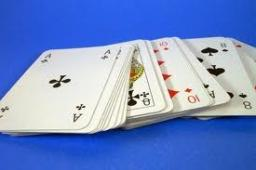Did you find an error or inaccuracy? Feel free to write us. Thank you!## Related math problems and questions:

• Unknown number 2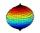I think the number. When he reduces it four times, I'll get 11. What number am I thinking?
• Numbers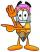By how many is the difference of numbers 8 and 34 less than its product?
• SelectSelect the statement that describes this expression: 5 x (3 + 4) - 4. a) 5 times the sum of 3 and 4, then subtract 4 b) 5 times 3 plus 4 minus 4 c) 5 more than the sum of 3 and 4, and then subtract 4 d) 5 times 3 plus the difference of 3 and 4 What is the
• Computer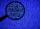A print line on a computer contains 64 characters (letters, spacers, or other chars). Find how many characters there are in 7 lines.
• 3 cats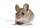3 cats eat 3 mice in 3 days. How many mice will be eaten by 10 cats in 10 days?
• Cakes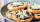On the table was 56 cakes. When Peter went home, leaving them eight times less. How many cakes left?
• Piggy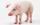A man bought a pig for € 60 then sold it for € 75. Then again bought for € 90 and sold it for € 100. How many € earned?Add marks (+, -, *, /, brackets) to fullfill equations 1 3 6 5 = 10 This is for the 4th grade of the primary school - with no negative numbers yet
• Chess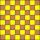How many ways can select 4 fields on a classic chessboard with 64 fields so that fields don't have the same color?
• SalamiHow many ways can we choose 5 pcs of salami if we have 6 types of salami for 10 pieces and one type for 4 pieces?
• Cents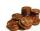Julka has 3 cents more than Hugo. Together they have 27 cents. How many cents has Julka and how many Hugo?
• WindowsCalculate the masonry area to build a wall with dimensions of 9 m × 4 m with 4 windows of size 64 cm × 64 cm.
• Children in the classroom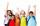How many children are in the classroom, where Ladislav is 10th lightest and 16th heaviest in class?
• Czech crownsOldrich has one crown. Peter has five crowns coin, a two crown coin and a one-crown coin. Radek has twenty-crown banknote, ten banknote and five-crown coin. The boys got one fifty-one crown and one crown coin. How can they share the money fairly when they
• ZOO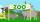In the zoo was elephants as many as ostrichs. Monkeys was 4 times more than elephants. Monkeys were as many as flamingos. Wolves were 5 times less as flamingos. How many of these animals were together? We know that there were four wolves.
• Page numberingThe book has 88 pages. How many times is the number 4 used for the book numbering?
• Rabbits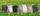In the hutch are 48 mottled rabbits. Brown are 23 less than mottled and white are 8-times less than mottled. How many rabbits are in the hutch?